Question

# Compute interest and find the maturity date for the following notes. (Use 360 days for calculation.)...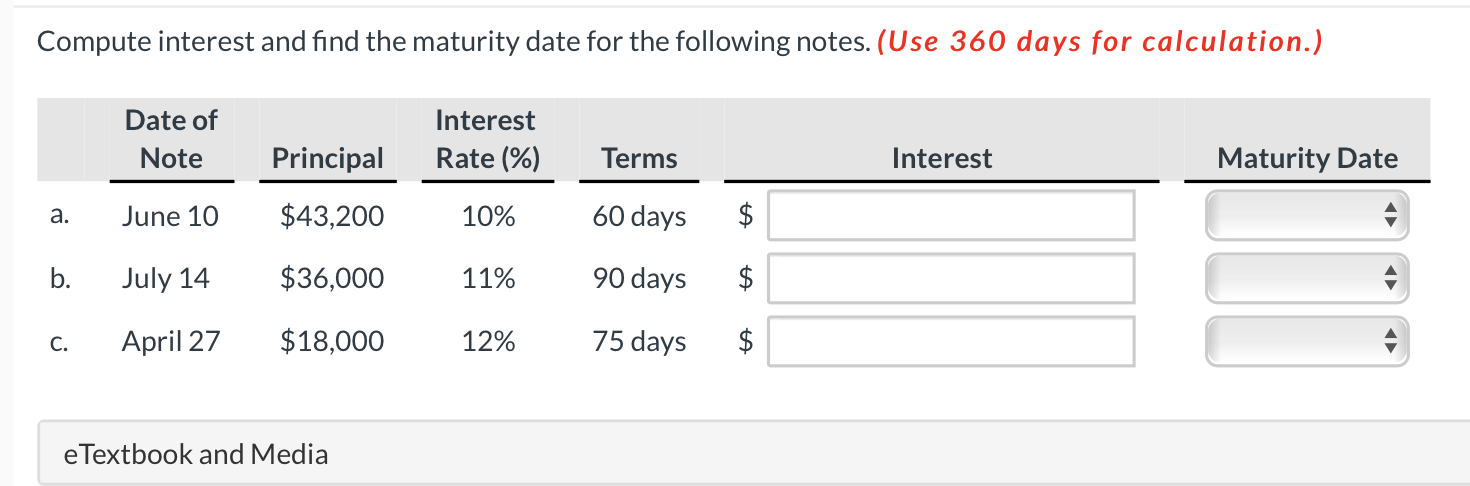Compute interest and find the maturity date for the following notes. (Use 360 days for calculation.) Date of Note Interest Rate (%) Principal Terms Interest Maturity Date a. June 10 \$43,200 10% 60 days \$ b. July 14 \$36,000 11% 90 days \$ C. April 27 \$18,000 12% 75 days \$ \$ e Textbook and Media

Interest = Principal * Rate * Time

Assumption : No. of days = 360 days

a) Interest = \$ 43200*10%*60/360 = \$ 720

Maturity = Days in June (30-10 = 20) + Days in July = 31+ Remaining days after June and July (69-20-31) = 9th August.

b) Interest = \$ 36000*11%*90/360 = \$ 990

Maturity = Days in July (31-14 = 17) + Days in August = 31+ Remaining days after September = 30+ Remaining days after Jult,August and September (90 - 17 -31 -30) = 12th October.

c) Interest = \$18000*12%*75/360 = \$450

Maturity = Days in April (30 - 27 = 3) + Days in May = 31 + Days in June = 30+ Remaining days after April, May and June (75 - 3 - 31 - 30) = 11th July.

#### Earn Coins

Coins can be redeemed for fabulous gifts.

Similar Homework Help Questions
• ### Compute interest and find the maturity date for the following notes. (Round answers to O decimal...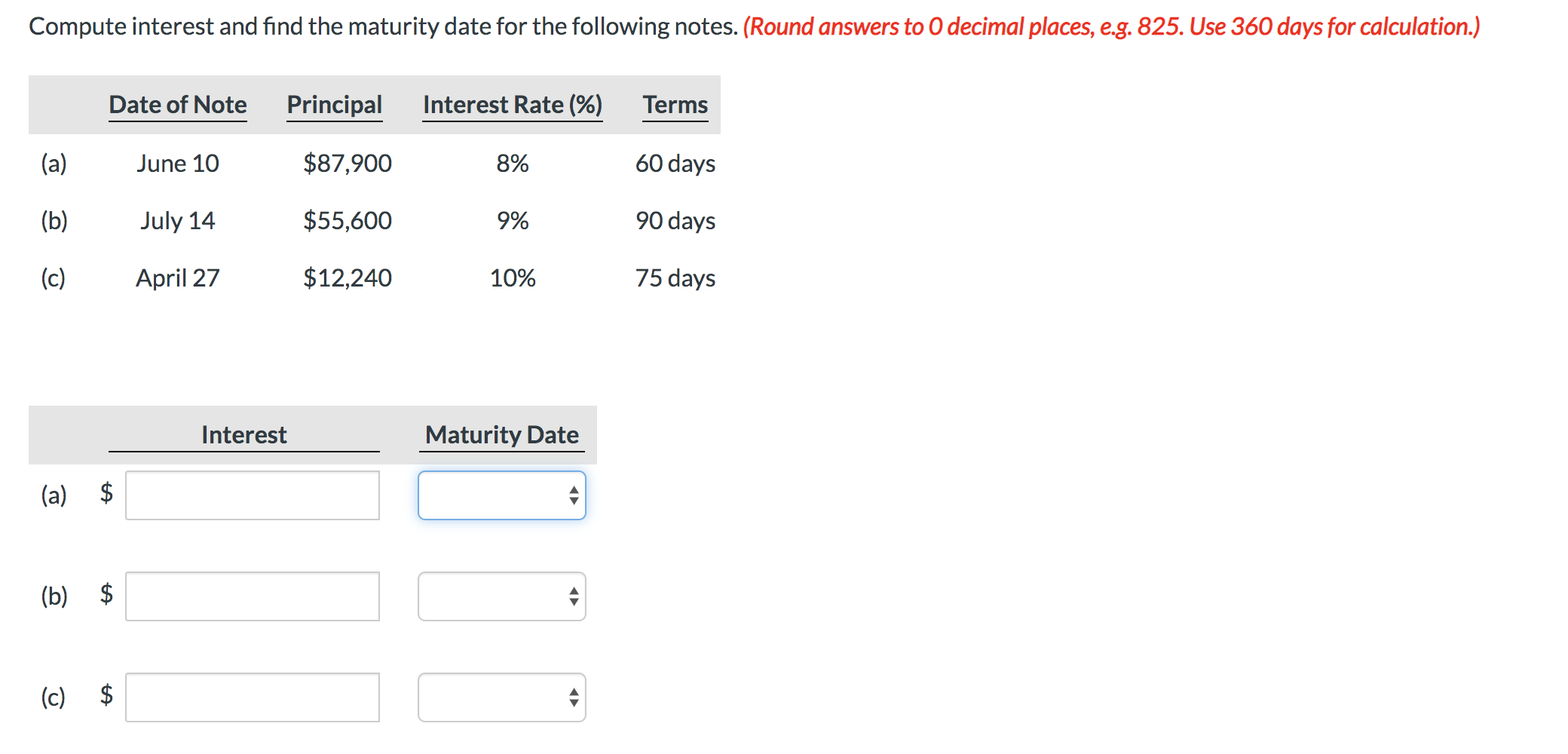Compute interest and find the maturity date for the following notes. (Round answers to O decimal places, e.g. 825. Use 360 days for calculation.) Date of Note Principal Interest Rate (%) Terms June 10 \$87,900 ཆུ 8% 60 days (b) July 14 \$55,600 9% 90 days April 27 \$12,240 10% 75 days Interest Maturity Date

• ### Compute interest and find the maturity date for the following notes. (Round answers to 0 decimal...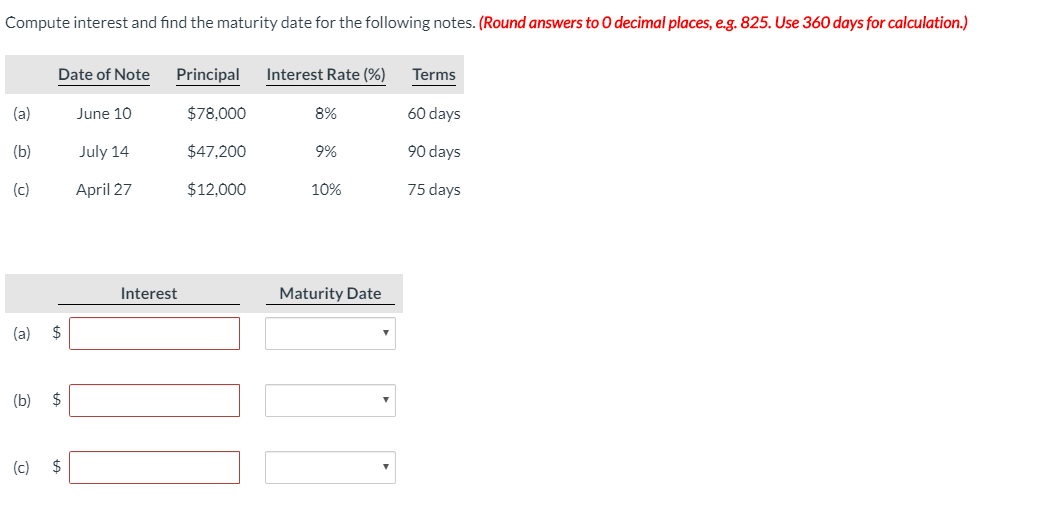Compute interest and find the maturity date for the following notes. (Round answers to 0 decimal places, eg. 825. Use 360 days for calculation.) Date of Note Principal Interest Rate (%) Terms June 10 \$78,000 8% 60 days \$47,200 9 % 90 days (b) (6) July 14 April 27 \$12,000 10% 75 days Interest Maturity Date (a) \$ (c) \$

• ### Your answer is partially correct. Compute interest and find the maturity date for the following notes....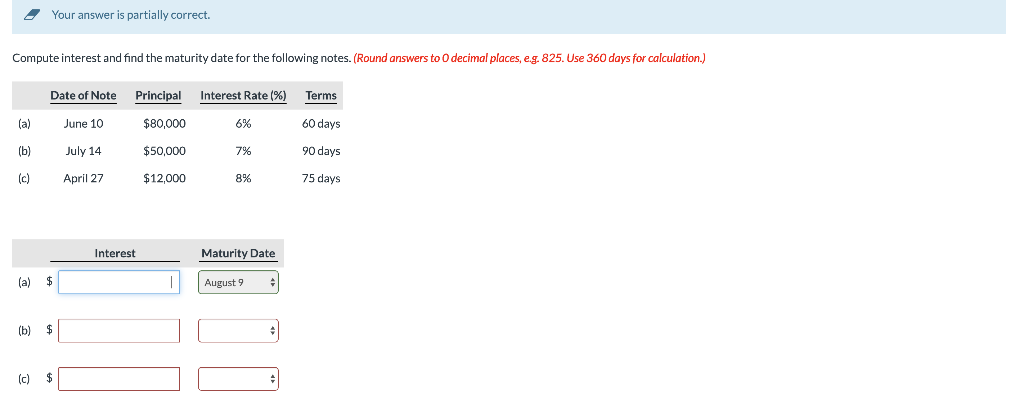Your answer is partially correct. Compute interest and find the maturity date for the following notes. (Round answers to O decimal places, e.g. 825. Use 360 days for calculation.) Date of Note Principal Interest Rate(%) Terms la) June 10 \$80,000 60 days \$50,000 90 days (b) (c) July 14 April 27 \$12,000 8% 75 days Interest Maturity Date (ə) \$ 1 August 9 %

• ### Compute interest and find the maturity date for the following notes. (Round answers to decimal places,...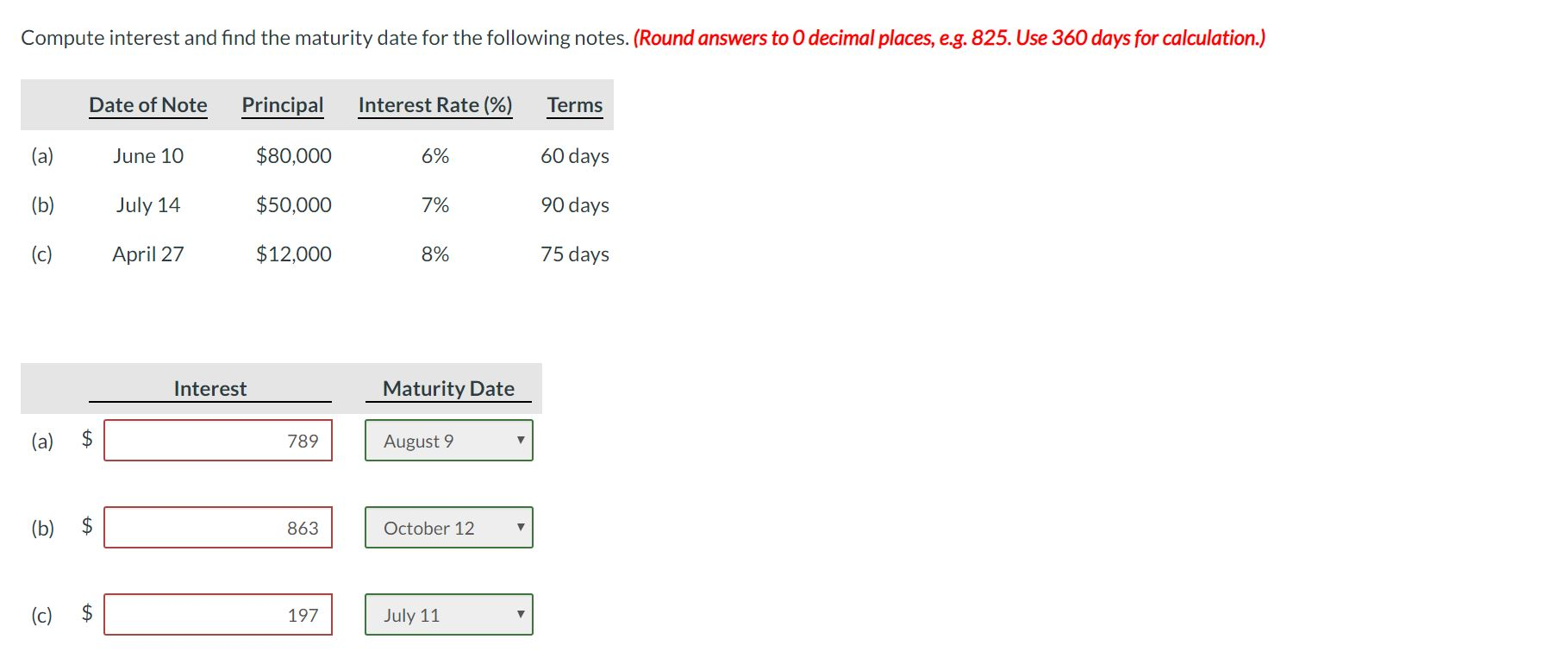Compute interest and find the maturity date for the following notes. (Round answers to decimal places, e.g. 825. Use 360 days for calculation.) Date of Note Principal Interest Rate (%) Terms (a) June 10 \$80,000 6% 60 days (b) July 14 \$50,000 7% 90 days (c) April 27 \$12,000 8% 75 days Interest Maturity Date (a) \$ 789 August 9 (b) \$ 780 863 97) 863 October 12 C \$ C 1 197 पी July 11 July 21

• ### Compute interest and find the maturity date for the following notes. (Round answers to O decimal...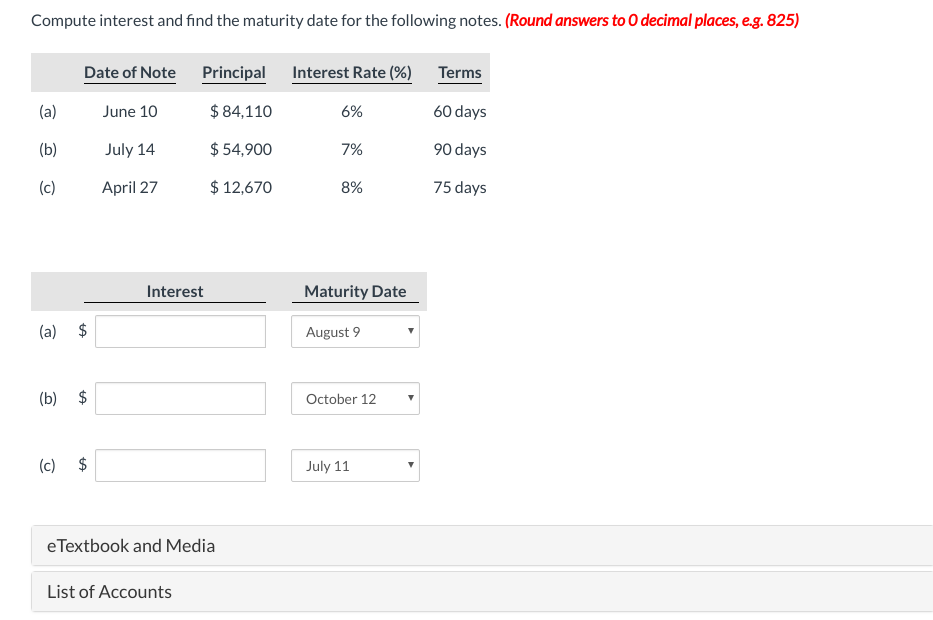Compute interest and find the maturity date for the following notes. (Round answers to O decimal places, eg. 825) Date of Note Principal (a) ne 10 (b) July 14 (c) April 27 \$ 84,110 54,900 \$12,670 Interest Rate (%) 6% 7% 8% Terms 60 days 90 days 75 days Interest Maturity Date (a) \$ August 9 (b) \$ October 12 (c) \$ July 11 eTextbook and Media List of Accounts

• ### Compute interest and find the maturity date for the following notes. (Round answers to 0 decimal...

Compute interest and find the maturity date for the following notes. (Round answers to 0 decimal places, e.g. 825. Use 360 days for calculation.) Date of Note Principal Interest Rate (%) Terms (a) June 10 \$75,600 6% 60 days (b) July 14 \$48,400 7% 90 days (c) April 27 \$12,840 8% 75 days Interest Maturity Date (a) \$enter the amount of interest in dollars rounded to 0 decimal places select a maturity date                       ...

• ### Maturity Dates of Notes Receivable Determine the maturity date and compute the interest for each of...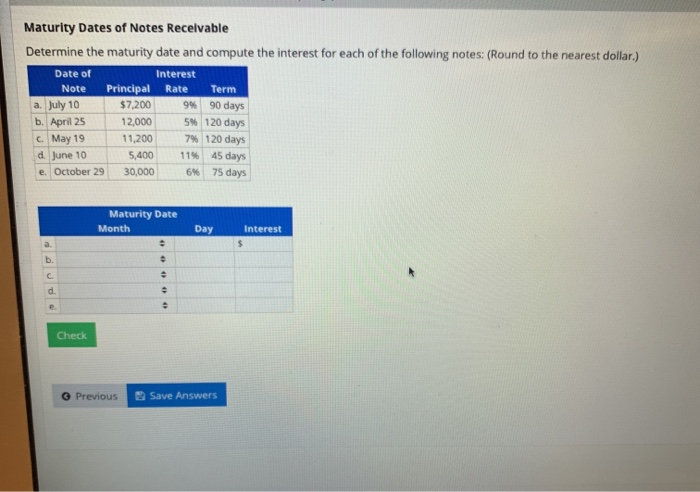Maturity Dates of Notes Receivable Determine the maturity date and compute the interest for each of the following notes: (Round to the nearest dollar.) Date of Interest Note Principal Rate Term a. July 10 \$7,200 94 90 days b. April 25 12,000 5 120 days May 19 11,200 7% 120 days d June 10 5.400 1145 days e. October 29 30,000 6% 75 days Maturity Date Month Day Interest Check Previous Save Answers

• ### Maturity Dates of Notes Receivable Determine the maturity date and compute the interest for each of...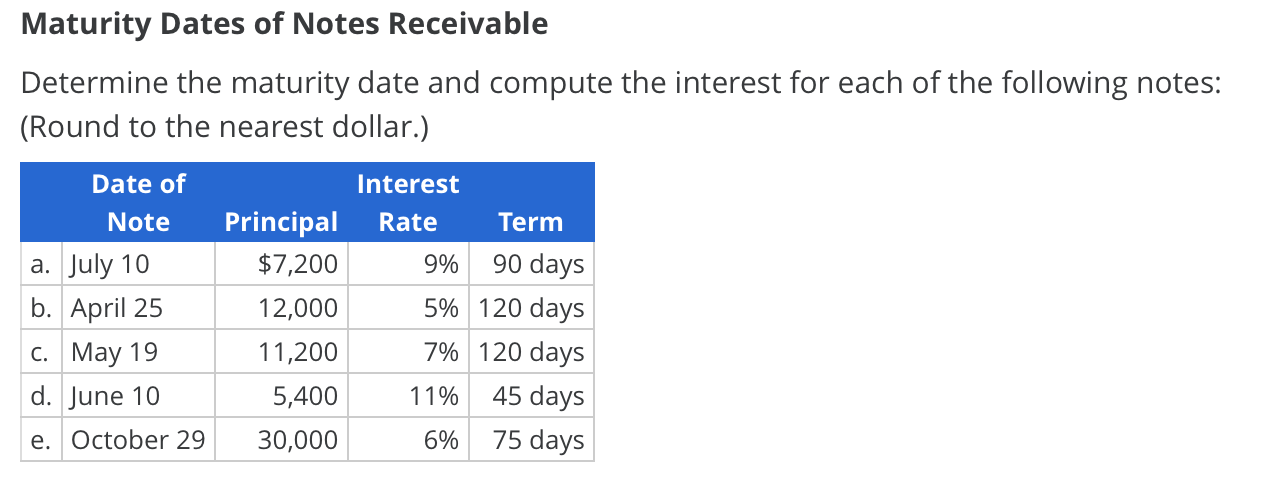Maturity Dates of Notes Receivable Determine the maturity date and compute the interest for each of the following notes: (Round to the nearest dollar.) Date of Interest Principal Rate Note Term 90 days \$7,200 а. July 10 9% 5% 120 days b. April 25 12,000 7% 120 days C. May 19 11,200 45 days d. June 10 5,400 11% 75 days e. October 29 30,000 6%

• ### Determine the maturity date and compute interest for each note. (Use 360 days a year. Do...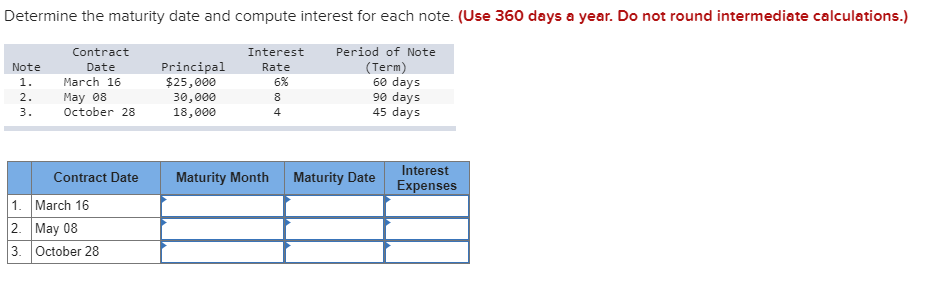Determine the maturity date and compute interest for each note. (Use 360 days a year. Do not round intermediate calculations.) Period of Note Contract Interest Principal \$25,000 (Term) 60 days 90 days 45 days Note Date Rate March 16 6% 1. May 08 October 28 2. 30,000 18,000 8 3. 4 Interest Maturity Month Maturity Date Contract Date Expenses 1. March 16 2. May 08 3 October 28

• ### Determine the maturity date and compute interest for each note. (Use 360 days a year. Do...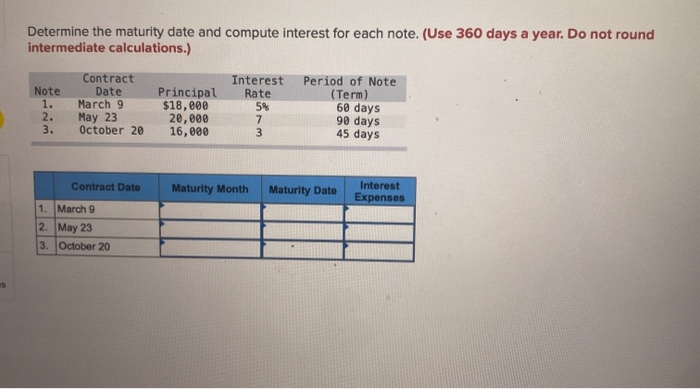Determine the maturity date and compute interest for each note. (Use 360 days a year. Do not round intermediate calculations.) Note 1. 2. 3. Contract Date March 9 May 23 October 20 Principal \$18,000 20,000 16,000 Interest Rate 5% 7 3 Period of Note (Term) 60 days 90 days 45 days Contract Date Maturity Month Maturity Dato Interest Expenses 1. March 9 2. May 23 3. October 20